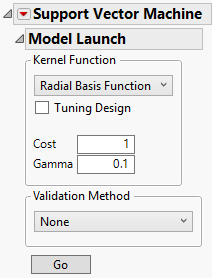Publication date: 11/10/2021

##Model Launch Control Panel

When you click OK in the launch window, the Support Vector Machine report window appears, showing a Model Launch control panel for fitting models. Use the Model Launch control panel to specify the kernel function and associated parameter values, as well as the validation method.

Figure 9.5 The Model Launch Control PanelThe Model Launch control panel contains the following options:

Kernel Function

Specifies the kernel function used in the model. Choose from the following kernel functions:

Selects the radial basis function kernel to create a nonlinear hyperplane to separate the classes.

The Cost parameter is the penalty associated with misclassifying an observation in the training set. A higher cost parameter implements an algorithm that is less likely to misclassify a point in the training set, whereas a lower cost parameter produces a wider margin. The Cost parameter must be greater than 0, and the default value is 1.

The Gamma parameter is the parameter in the kernel function. This parameter determines the amount of curvature there is to the decision line; a higher Gamma value indicates more curvature. A nonlinear decision line provides a more flexible fit, but too much curvature can lead to overfitting. The Gamma parameter must be greater than 0, and the default value is 1/(# of predictors).

Linear

Selects the linear kernel function to create a linear hyperplane to separate the classes.

The Cost parameter is the penalty associated with misclassifying an observation in the training set. A higher cost parameter implements an algorithm that is less likely to misclassify a point in the training set, whereas a lower cost parameter produces a wider margin. The Cost parameter must be greater than 0, and the default value is 1.

Note: If you specify parameter values that are out of range, the default values are used.

Tip: To find the best fitting model, fit a range of kernel functions and parameter values and use the Model Comparison report.

Tuning Design

Enables you to fit a range of parameter values for the specified kernel. The models with the largest RSquare and the smallest Misclassification Rate or RASE are identified in the Model Comparison report. After you select Tuning Design, you must specify minimum and maximum values for the parameters. Default values are provided based on the data and the minimum must be greater than zero. You must also specify a value for the Number of Runs. The SVM platform fits that many models over a grid of parameter values determined by the minimum and maximum values.

Validation Method

Specifies the model validation method. When you click the Go button for the first time, the first SVM model is fit using the specified validation method. This Validation Method is then used for all SVM models fit from within the SVM window. This ensures that all models in the report window are fit using the same validation method and validation set.

Holdback

Randomly divides the original data into training and validation sets. You can specify the proportion of the original data to use as the validation set (holdback).

KFold

(Available only when Y is continuous or nominal.) Randomly divides the original data into K subsets. In turn, each of the K sets is used to validate the model fit on the rest of the data, fitting a total of K models. If Y is continuous, the model that has the best validation RASE statistic is chosen as the final model. If Y is nominal, the model that has the best validation misclassification rate is chosen as the final model.

Validation Column

(Available only if you specified a Validation column in the launch window.) Uses the values in the specified Validation column to divide the data into parts. The column’s values determine how the data are split, and what method is used for validation:

If there are two values, the smaller value defines the training set and the larger value defines the validation set.

If there are three values, these values define the training, validation, and test sets in order of increasing size.

If the validation column has more than three levels, then Validation Column K Fold is used.

The SVM platforms uses the validation column to train and evaluate the model, unless a Tuning Design is used. If the Tuning Design option is selected, the SVM platform uses the validation column to train and tune the model or to train, tune, and evaluate the model. For more information about validation, see Validation in JMP Modeling.

Note: If the validation column does not lead to a valid partition of the data, the Holdback validation method is used instead.

Validation Column K Fold

(Available only when the Y, Response column has exactly two levels and a Validation column is specified in the launch window.) Uses the values in the specified Validation column to divide the data into K sets, where K is the number of unique values in the column. Then, K-Fold validation is performed.

None

No validation used.

Go

Fits the specified SVM model and shows the model report.

Note: If you have a large data table, a progress bar is shown for each model that is fit to the data. The total number of models fit is k!/2(k-2)!, where k is the number of levels of the response variable. Each progress bar has an Accept Current Estimates button. Click this button if you want to stop the fitting algorithm early and accept the current estimates. Because prediction calculations are performed after you click this button, it may take some time for the report to appear.

#### Missing Values

Rows that contain any missing predictor values are not included in the SVM modeling procedure. Therefore, any columns saved to the data table will contain missing values in those rows. If you want to include data with missing values in an SVM model, some form of preprocessing is required. See Explore Missing Values Utility.